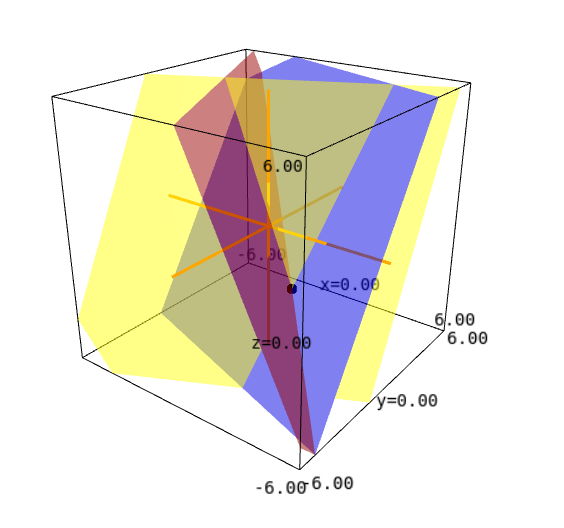# Sage won't plot more than two plains

Hello, Sage community!

I have the following code that shows the solution of a 3x3 system of equations as an interact:

A = matrix(RR, 3, 3, [2,1,1,1,-2,1,1,3,-2] )
var('x y z')

@interact
def sol_3ecu_3inc(k1 = slider(-5, 5, 0.1, -1), k2 = slider(-5, 5, 0.1, -2), k3 = slider(-5, 5, 0.1, -3)):

bvec = vector(RR, [k1, k2, k3])
xvec = A \ bvec

augmented_matrix = A.augment(bvec, subdivide=True)

myplot += point3d((xvec, xvec, xvec), color='black', size=100)

show(myplot)


The result should look like this:When I run this code in CoCalc or my own SageMath local installation, it works perfectly. However, if I try to execute this in sagecell.sagemath.org, Sage won't be willing to plot this. However, if I delete one the implicit_plot3d commands, the plot magically appears.

Can somebody explain this? Is this reproducible for other users?

edit retag close merge delete

Sort by » oldest newest most votedConcerning the second question, yes, it is reproducible. It seems that SageCell only plots one or two implicit_plot3d. I've been experimenting with this code:

var("x,y,z")
fig = implicit_plot3d(x^2+y+z==0, (x,-1,1), (y,-1,1), (z,-1,1), color="red")
#fig += implicit_plot3d(x-y+z^2==0, (x,-1,1), (y,-1,1), (z,-1,1), color="green")
#fig += implicit_plot3d(x-y^2+z^2==0, (x,-1,1), (y,-1,1), (z,-1,1), color="blue")
show(fig)
print("Finished")


Sometimes I could only plot one surface, sometimes two, but never the three surfaces.

more

1

I wonder why this is happening. This is quite strange. Could this be a bug or a problem of SageCell itself?